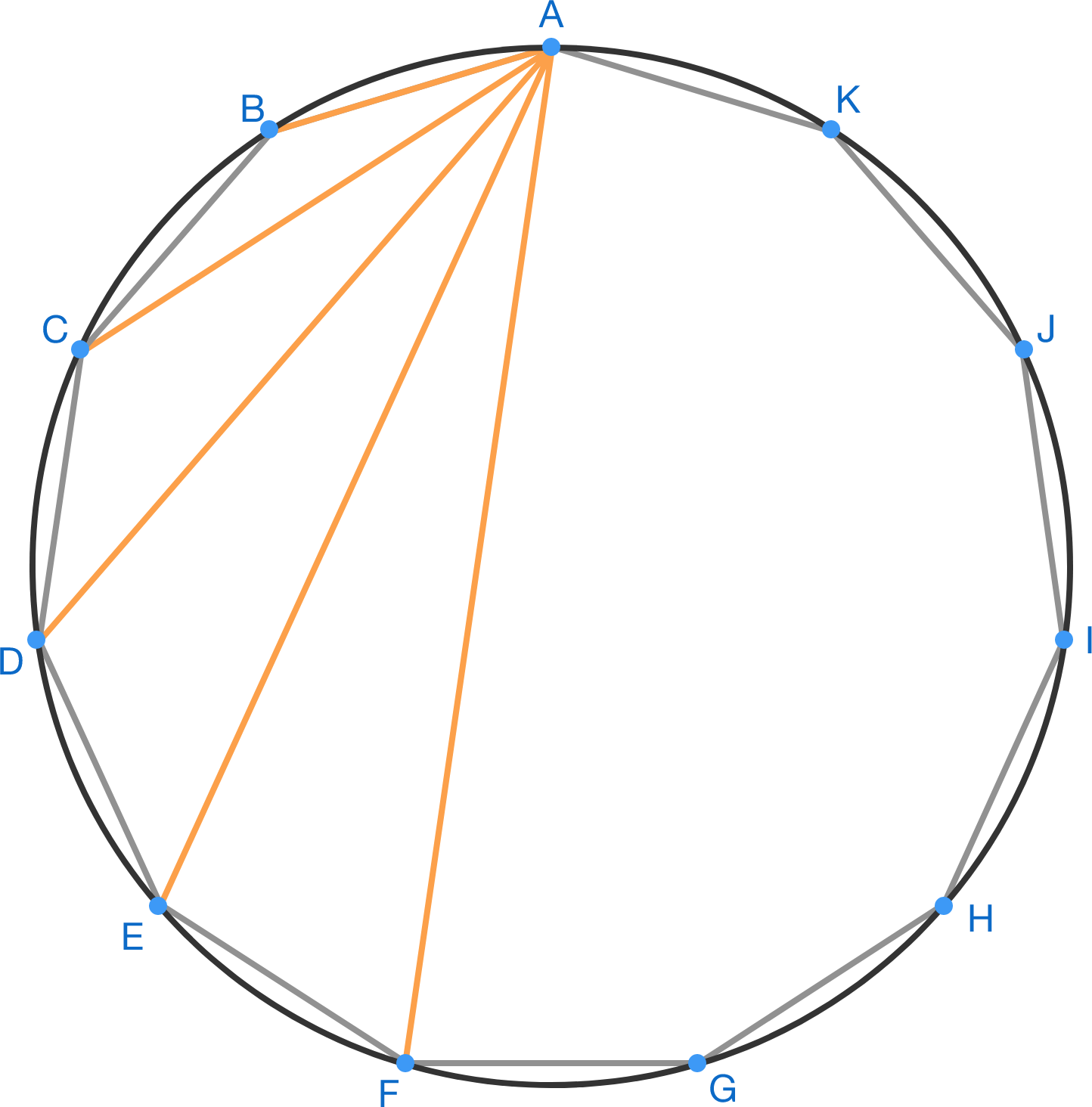# New Year's Countdown Day 11: Undecagon

Geometry Level 4

A regular 11-sided polygon $ABCDEFGHIJK$ is inscribed in a unit circle, as shown below.

If $AB \times AC \times AD \times AE \times AF = \sqrt{k}$

for some positive integer $k,$ find the sum of digits of $k.$×